# Production function

--
23 de Mar de 2018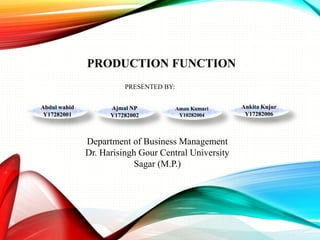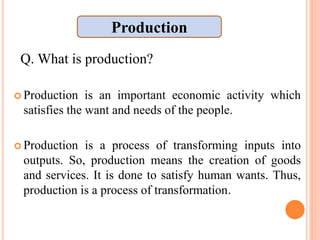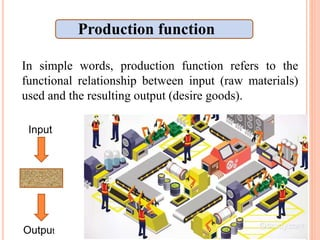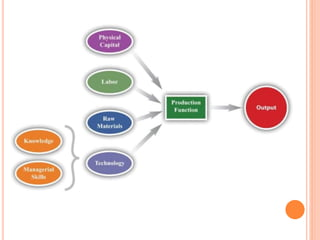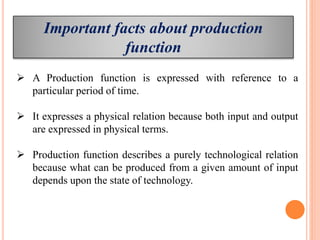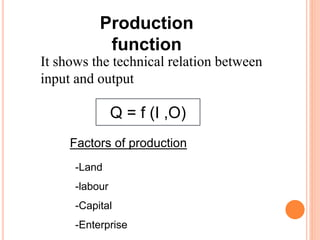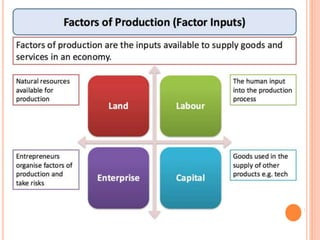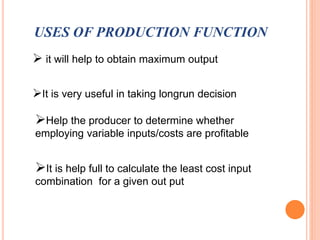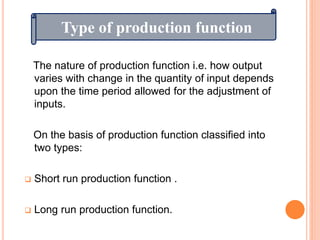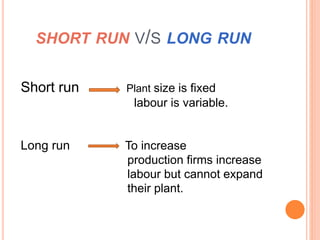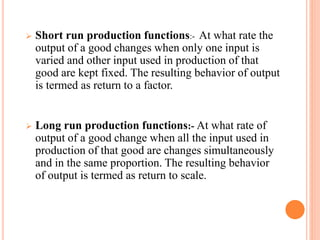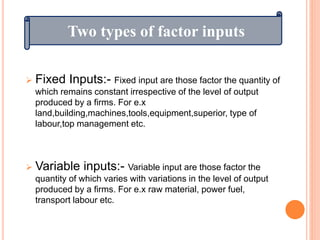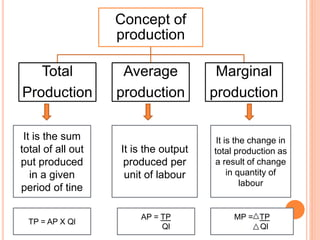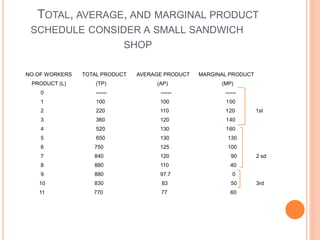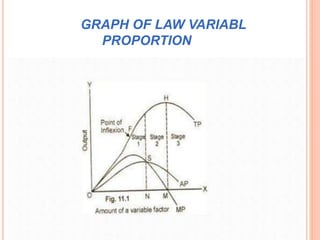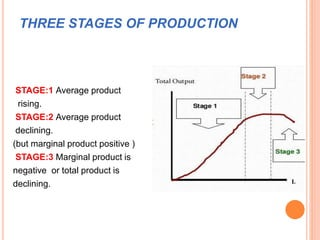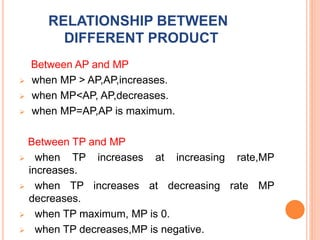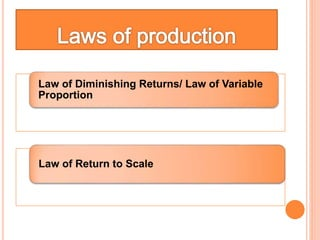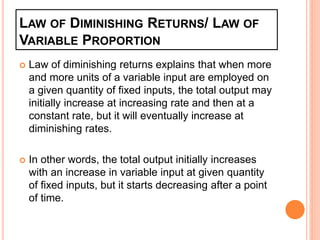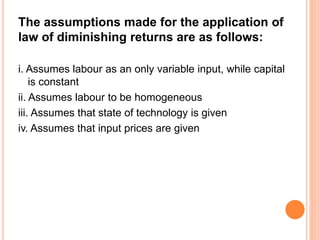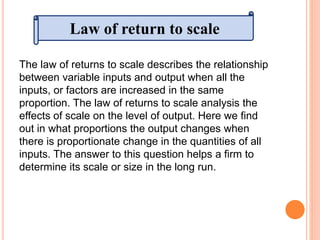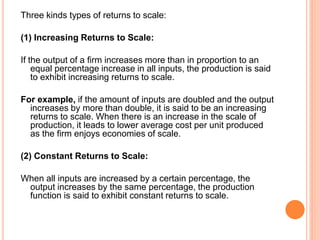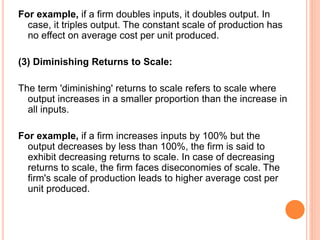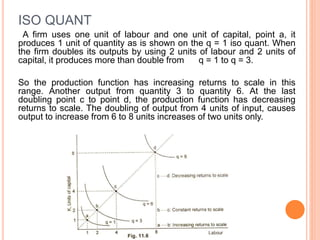1 de 25

### Production function

• 1. PRODUCTION FUNCTION Abdul wahid Y17282001 Ajmal NP Y17282002 Aman Kumari Y10282004 Ankita Kujur Y17282006 Department of Business Management Dr. Harisingh Gour Central University Sagar (M.P.) PRESENTED BY:
• 2.  Production is an important economic activity which satisfies the want and needs of the people.  Production is a process of transforming inputs into outputs. So, production means the creation of goods and services. It is done to satisfy human wants. Thus, production is a process of transformation. Q. What is production? Production
• 3. In simple words, production function refers to the functional relationship between input (raw materials) used and the resulting output (desire goods). Output Input Production function
• 5. Important facts about production function  A Production function is expressed with reference to a particular period of time.  It expresses a physical relation because both input and output are expressed in physical terms.  Production function describes a purely technological relation because what can be produced from a given amount of input depends upon the state of technology.
• 6. Production function It shows the technical relation between input and output Q = f (I ,O) Factors of production -Land -labour -Capital -Enterprise
• 8. USES OF PRODUCTION FUNCTION  it will help to obtain maximum output Help the producer to determine whether employing variable inputs/costs are profitable It is help full to calculate the least cost input combination for a given out put It is very useful in taking longrun decision
• 9. The nature of production function i.e. how output varies with change in the quantity of input depends upon the time period allowed for the adjustment of inputs. On the basis of production function classified into two types:  Short run production function .  Long run production function. Type of production function
• 10. SHORT RUN V/S LONG RUN Short run Plant size is fixed labour is variable. Long run To increase production firms increase labour but cannot expand their plant.
• 11.  Short run production functions:- At what rate the output of a good changes when only one input is varied and other input used in production of that good are kept fixed. The resulting behavior of output is termed as return to a factor.  Long run production functions:- At what rate of output of a good change when all the input used in production of that good are changes simultaneously and in the same proportion. The resulting behavior of output is termed as return to scale.
• 12.  Fixed Inputs:- Fixed input are those factor the quantity of which remains constant irrespective of the level of output produced by a firms. For e.x land,building,machines,tools,equipment,superior, type of labour,top management etc.  Variable inputs:- Variable input are those factor the quantity of which varies with variations in the level of output produced by a firms. For e.x raw material, power fuel, transport labour etc. Two types of factor inputs
• 13. Concept of production Total Production Average production Marginal production It is the sum total of all out put produced in a given period of tine It is the output produced per unit of labour It is the change in total production as a result of change in quantity of labour TP = AP X Ql AP = TP Ql MP = TP Ql
• 14. TOTAL, AVERAGE, AND MARGINAL PRODUCT SCHEDULE CONSIDER A SMALL SANDWICH SHOP NO.OF WORKERS TOTAL PRODUCT AVERAGE PRODUCT MARGINAL PRODUCT PRODUCT (L) (TP) (AP) (MP) 0 ------ ------ ------ 1 100 100 100 2 220 110 120 1st 3 360 120 140 4 520 130 160 5 650 130 130 6 750 125 100 7 840 120 90 2 sd 8 880 110 40 9 880 97.7 0 10 830 83 50 3rd 11 770 77 60
• 15. GRAPH OF LAW VARIABL PROPORTION
• 16. THREE STAGES OF PRODUCTION STAGE:1 Average product rising. STAGE:2 Average product declining. (but marginal product positive ) STAGE:3 Marginal product is negative or total product is declining.
• 17. RELATIONSHIP BETWEEN DIFFERENT PRODUCT Between AP and MP  when MP > AP,AP,increases.  when MP<AP, AP,decreases.  when MP=AP,AP is maximum. Between TP and MP  when TP increases at increasing rate,MP increases.  when TP increases at decreasing rate MP decreases.  when TP maximum, MP is 0.  when TP decreases,MP is negative.
• 18. Law of Diminishing Returns/ Law of Variable Proportion Law of Return to Scale
• 19. LAW OF DIMINISHING RETURNS/ LAW OF VARIABLE PROPORTION  Law of diminishing returns explains that when more and more units of a variable input are employed on a given quantity of fixed inputs, the total output may initially increase at increasing rate and then at a constant rate, but it will eventually increase at diminishing rates.  In other words, the total output initially increases with an increase in variable input at given quantity of fixed inputs, but it starts decreasing after a point of time.
• 20. The assumptions made for the application of law of diminishing returns are as follows: i. Assumes labour as an only variable input, while capital is constant ii. Assumes labour to be homogeneous iii. Assumes that state of technology is given iv. Assumes that input prices are given
• 21. The law of returns to scale describes the relationship between variable inputs and output when all the inputs, or factors are increased in the same proportion. The law of returns to scale analysis the effects of scale on the level of output. Here we find out in what proportions the output changes when there is proportionate change in the quantities of all inputs. The answer to this question helps a firm to determine its scale or size in the long run. Law of return to scale
• 22. Three kinds types of returns to scale: (1) Increasing Returns to Scale: If the output of a firm increases more than in proportion to an equal percentage increase in all inputs, the production is said to exhibit increasing returns to scale. For example, if the amount of inputs are doubled and the output increases by more than double, it is said to be an increasing returns to scale. When there is an increase in the scale of production, it leads to lower average cost per unit produced as the firm enjoys economies of scale. (2) Constant Returns to Scale: When all inputs are increased by a certain percentage, the output increases by the same percentage, the production function is said to exhibit constant returns to scale.
• 23. For example, if a firm doubles inputs, it doubles output. In case, it triples output. The constant scale of production has no effect on average cost per unit produced. (3) Diminishing Returns to Scale: The term 'diminishing' returns to scale refers to scale where output increases in a smaller proportion than the increase in all inputs. For example, if a firm increases inputs by 100% but the output decreases by less than 100%, the firm is said to exhibit decreasing returns to scale. In case of decreasing returns to scale, the firm faces diseconomies of scale. The firm's scale of production leads to higher average cost per unit produced.
• 24. ISO QUANT A firm uses one unit of labour and one unit of capital, point a, it produces 1 unit of quantity as is shown on the q = 1 iso quant. When the firm doubles its outputs by using 2 units of labour and 2 units of capital, it produces more than double from q = 1 to q = 3. So the production function has increasing returns to scale in this range. Another output from quantity 3 to quantity 6. At the last doubling point c to point d, the production function has decreasing returns to scale. The doubling of output from 4 units of input, causes output to increase from 6 to 8 units increases of two units only.